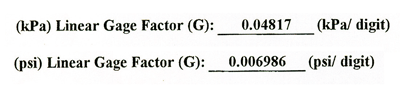# Sample Vibrating Wire Calculation

## Sample Calculation

Converting a VWT reading from the GK-404 to a depth to water

In our example, let's assume the VWT is connected to a monitoring port that is located at a depth of 100 ft (30.48 m) below ground surface.

Zero Reading (pre installation) of VWT: 9978 digits

Submerged Reading of VWT: 7978 digits

## From the "Vibrating Wire Pressure Transducer Calibration Report"

(sample in Appendix III), locate the "Linear Gage Factor" for each specific transducer serial number. In our example:To calculate the pressure head (psi or kPa) on the VWT, multiply the appropriate "Linear Gage Factor", by the difference between the zero reading and submerged reading. From our example:

0.006986 (psi/digit) x 2000 digits = 13.972 psi (96.33 kPa)

Therefore, VWT serial number 06-5822 has a pressure head of 13.972 psi (96.33 kPa).

To calculate the depth to water, simply convert psi (kPa) to feet (meters):

13.972 psi x 2.307 foot of head/psi = 32.23 feet of head.

96.33 kPa x 0.1020 meter of head/kPa = 9.826 meters of head

Now subtract the depth to the Port inlet (100ft/30.48m) from the pressure head.

100 ft - 32.23 feet of head = 67.77 ft.

30.48 m - 9.826 meters of head = 20.65 m